# How do I input a linear equation using the built-in template "ax + b = c"?

Select the "ax + b = c" format in the Settings window.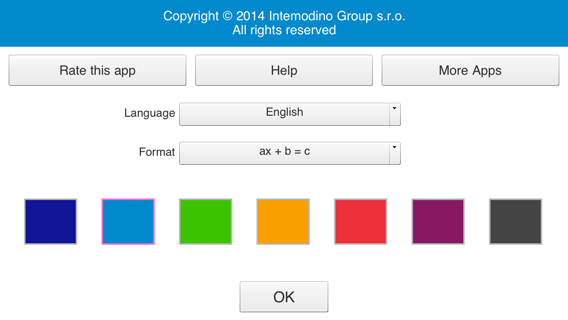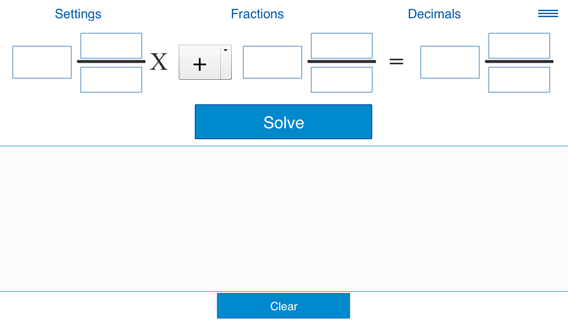Note: This window is available in the landscape orientation only.

Specify a, b and c. When you are done entering an equation, click Solve.

a, b and c could be whole numbers, fractions or decimals.

This format supports two modes: "Fractions" (coefficients could be whole numbers and fractions) and "Decimals" (coefficients could be whole numbers or decimals). Pressing "Fractions" and "Decimals" switches between the two modes.

The following examples show how to input equations.

Example 1. Solving the linear equation
4x - 15 = 45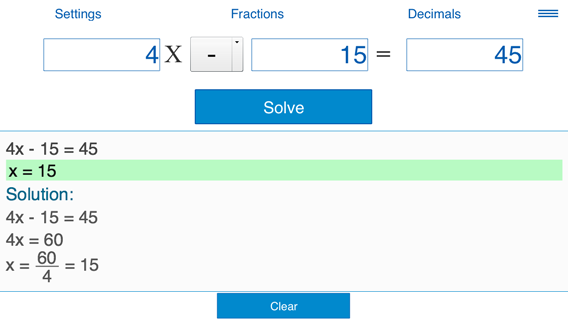Example 2. Solving the equation
-2x + 3.4 = 10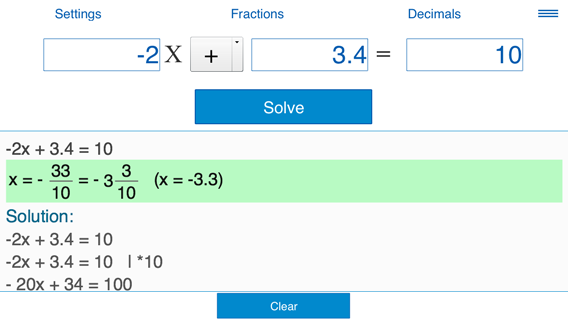Example 3. Solving the equation
-0.5x = 10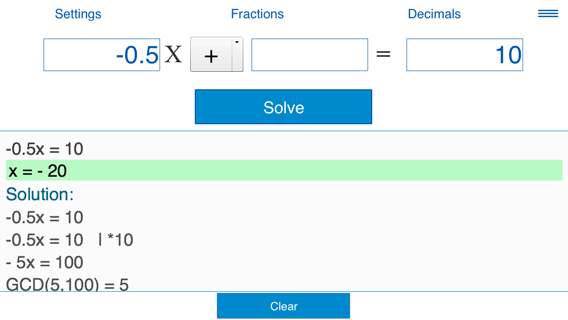Example 4. Solving the equation
1/2
x - 1
1/2
= -
1/4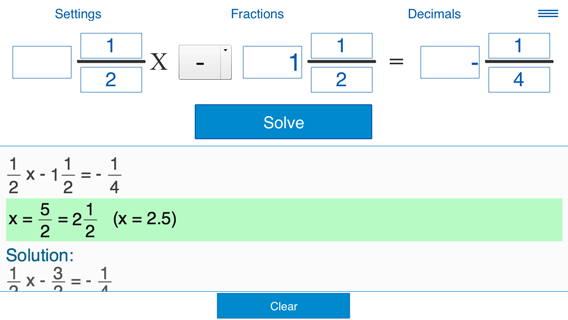Friday, September 22, 2023

Contact